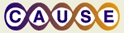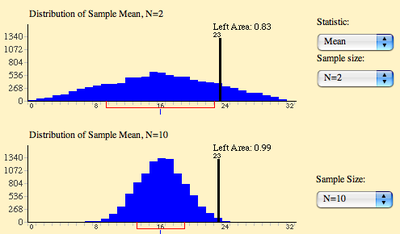# Simulating the Effect of Sample Size on the Sampling Distribution of the MeanThis material was originally developed through CAUSE
as part of its collaboration with the SERC Pedagogic Service.

#### SummaryThis activity allows students to explore the relationship between sample size and the variability of the sampling distribution of the mean. Students use a Java applet to specify the shape of the "parent" distribution and two sample sizes. The simulation then samples from the parent distribution to approximate the sampling distributions for the two sample sizes. Students can see both sampling distributions at the same time making them easy to compare. The activity also allows students to determine the probability of extreme sample means for the different sample sizes so that they can discover that small sample sizes are much more likely than large samples to produce extreme values.

Keywords: sampling distribution, sample size, simulation

## Learning Goals

Students should learn that the sampling distribution of the mean has much less variability with large sample sizes than with small sample sizes. They should also learn that extreme outcomes are less likely with large than with small samples. Students who begin the activity with a somewhat hazy concept of what a sampling distribution is should attain a better grasp of this essential concept.

## Context for Use

Students should be familiar with the concept of a sampling distribution before doing this activity.

This activity works well when each student has access to a computer, but can also be used when students share a computer and/or there is one computer with a screen projected for the whole class to see. This activity can be used with high school and college students. If done carefully, it can be adapted for middle school children.

Time involved:
5 minutes to introduce the activity
15 minutes to work, either individually or in groups
15 minutes for discussion and application of the concepts.

## Description and Teaching Materials

The simulation begins by showing a uniform "parent distribution" and is set to show the sampling distribution of the mean for sample sizes of 2 and 10. The parent distribution can be set to a normal distribution and sample sizes of 1, 2, 5, 10, 15 and 25 can be used. Students can experiment with the simulation as they see fit. However, since it is often difficult to choose a productive set of simulations, students can follow a set of step by step instructions that lead them to use the simulation to see the critical concepts. Students can determine the proportion of the distribution larger than a given value by "dragging" a vertical bar on the distribution to that value. This makes it easy to compare two distributions with regard to the proportion of means larger than a given value.

A set of questions is available for students to attempt to answer before they interact with the simulation. No feedback is given the first time students answer the questions other than an overall score. They are then asked to use the simulation to help them discover the answers to the questions. The second time through the questions feedback and explanations are given.

The simulation is available at http://onlinestatbook.com/chapter7/SampDist_v2.html. It can be downloaded for local from http://onlinestatbook.com.

## Teaching Notes and Tips

Students should be encouraged to answer the three questions presented in the simulation before they try out the simulation. It is a good idea to inform the students that they are not expected to know the answers to the questions, but should just make their best guesses. Then they should use the simulations to help them answer the questions.

The simulation provides general instructions and step-by-step instructions. It is preferable for the students to use only the general instructions. However, if they get stuck they should refer to the step-by-step instructions.

More advanced students may wish to contrast the effect of sample size on the sampling distribution of the range with the sampling distribution of the mean.

Following the work on the simulation it can be valuable to discuss the general principle that extreme outcomes are more likely with small sample sizes than with large sample sizes. For example, students can discuss whether it is more likely that a sample of two people or a sample of five people would have a mean height over six feet.

## Assessment

The questions offer some built-in assessment. Students may be asked to transfer the knowledge to new situations. Once classic problem that may be helped by going through this activity is as follows:

For a period of one year, two hospitals, the larger one having about 45 births per day and the smaller about 15 births per day, recorded the days on which more than 60% of the babies born were boys (given a gender ratio of 50:50). Participants were asked which hospital recorded more such days.

Since transfer of learning can be difficult, don't expect particularly good performance on this question. You may wish to introduce this problem (without discussion) before introducing the activity.

How is the variability of the sampling distribution of the mean affected by sample size?

How does sample size affect the probability that a sample mean will be extremely far from the population mean?

## References and Resources

The simulation is available at http://onlinestatbook.com/chapter7/SampDist_v2.html.